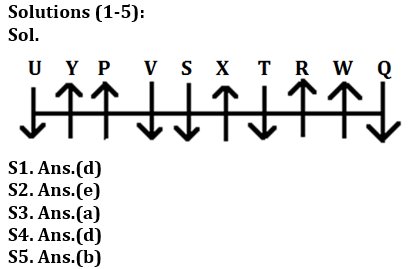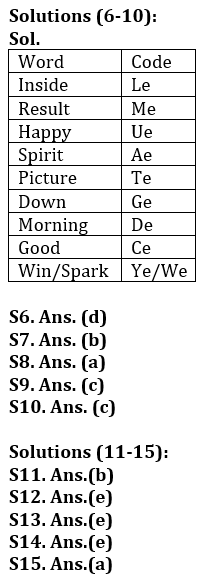Latest Banking jobs   »

# Reasoning Ability Quiz For LIC ADO Prelims 2023- 10th March

Directions (1-5): Study the information and answer the following questions:
Ten persons are sitting in a row some are facing north, and some are facing south (but not necessarily in the same manner). Not more than two persons facing same direction sits together.
Five persons sit between P and W and both faces same direction. Neither P nor W sits at any end. U sits second to the left of P. Q is an immediate neighbor of W. S sits fourth to the left of U and both faces same direction. T sits third to the right of Q and faces same direction as Q. Y who faces north is not a neighbor of S and T. X sit second to the left of R and both faces same direction. U is facing south direction. V neither face same direction as R nor is an immediate neighbor of W.

Q1. Who among the following sits to the immediate right to T?
(a) S
(b) W
(c) U
(d) X
(e) None of these

Q2. How many persons sit between Q and V?
(a) One
(b) Four
(c) Two
(d) Three
(e) More than four

Q3. Four of the following five are alike in a certain way and form a group, who among the following does not belongs to that group?
(a) Q
(b) X
(c) Y
(d) W
(e) P

Q4. Which among the following pair sits at the extreme ends of the row?
(a) P, U
(b) S, X
(c) Q, X
(d) U, Q
(e) None of these

Q5. How many persons face south?
(a) Four
(b) Five
(c) Three
(d) One
(e) Two

Directions (6-10): Read the following information carefully and answer the questions given below.
In a certain code language:
“Inside result happy spirit” is written as “ae me le ue”.
“Picture down morning inside” is written as “de le te ge”.
“Happy picture good spirit” is written as “te ae ue ce”.
“Spirit win morning spark” is written as “de ye we ae”.

Q6. What is the possible code for “good way down”?
(a) ce me ge
(b) pe me ge
(c) ce te le
(d) pe ce ge
(e) None of these

Q7. If “Inside win picture” is coded as “ye le te”, then what will be the code for “Spark”?
(a) xe
(b) we
(c) ce
(d) de
(e) Can’t determined

Q8. What is the code for “Morning”?
(a) de
(b) te
(c) ae
(d) ue
(e) None of these

Q9. What is the word for the code “me” in the given code language?
(a) spirit
(b) good
(c) result
(d) spark
(e) win

Q10. If “Spirit give happy” is written as “ue ae re”, then what will be the code for “Give”?
(a) ae
(b) ue
(c) re
(e) Can’t determine

Directions (11-15): Answer these questions referring to the letter sequence given below:

O P H Z A R S N I J K U V G Q Y B F E L M T W X D C

Q11. If letters of the above given series are written in reverse order, then which letter will be third to the left of eighteenth letter from right?
(a) Z
(b) M
(c) I
(d) L
(e) None of these

Q12. Which of the following letter is 3rd to left of 6th from left?
(a) S
(b) A
(c) I
(d) F
(e) H

Q13. Which of the following is the fifth to the right of thirteenth letter from left?
(a) U
(b) J
(c) S
(d) Z
(e) None of these

Q14. Which of the following is 5th to right of 3rd from left?
(a) C
(b) Q
(c) K
(d) Y
(e) N

Q15. Which of the following letter is 4th to right of 8th from right?
(a) W
(b) T
(c) X
(d) E
(e) P

Solutions## FAQs

### When will the LIC AAO Prelims Exam 2023 be conducted?

The LIC AAO Prelims Exam 2023 will be conducted on 17th & 20th February 2023.

#### Congratulations!Union Budget 2023-24: Free PDF